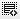## how to drop duplicate rows in pandas0

Hello,

I have duplicate rows in data, let's say  'b' is the unique Id of the table

``````df = pd.DataFrame({
'a': [1,2,3,4,5, 5, 5],
'b': ['a', 'e', 'b', 'c', 'd', 'd', 'd'],
'c': [False, True, True, False, True, True, True],
'd': [2006, 2006, 2007, 2008, 2006, 2006, 2007]
})

df``````
a b c d
0 1 a False 2006
1 2 e True 2006
2 3 b True 2007
3 4 c False 2008
4 5 d True 2006
5 5 d True 2006
6 5 d True 2007

In the above data, row 4,5, 6 are similar. row 4 & 5 are exactly similar so if i use  "df.drop_duplicates()"  either 4 or 5 is removed but 6 is not removed because column 'd' has different values. So should I:

a) drop column 'd' and then run "df.drop_duplicates()", this way will loose column 'd' or

b) should I create another column and concatenate the values in column 'd' as '2006|2007' and then run "df.drop_duplicates()". This seems resonable but I dont know how to concatenate column values from two similar rows? Can you please help.

Or is there is better way to acheive such kind of duplicate data?0

By definition of duplicates, only row index 4 and 5 are duplicates. However snce you need to find duplicates as per only column b and c, you can perform a groupby on b and c and then convert the rows that you get as a single row.

Here are some alternatives based on what you need. Check the difference in the column d to understand what is happening. (Also note that you lose the relavance of the index).

``df.groupby(['b', 'c']).max().reset_index()``
``df.groupby(['b', 'c']).min().reset_index()``
``````group_cols = ['b', 'c']
other_cols = [c for c in df.columns if c not in group_cols]
df.groupby(group_cols).apply(lambda d: d[other_cols].apply(lambda var2: '|'.join([str(v) for v in var2.value_counts().index])))``````

Click on thisicon to add code snippet.